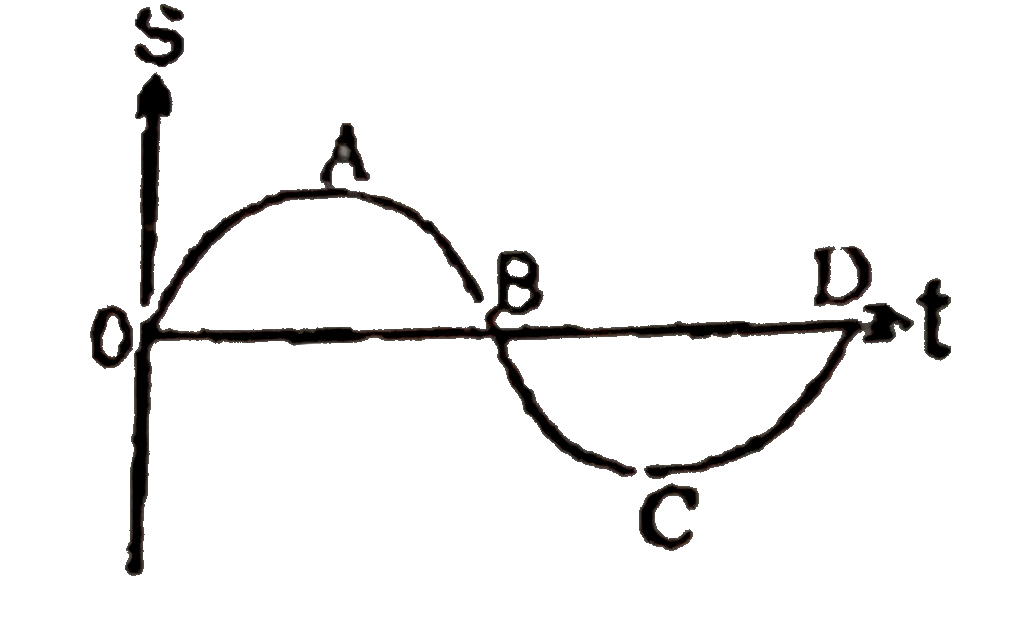Doubtnut App - Get 50 Lakh+ video solutions better than GoogleInstall Now

# A particle has a rectilinear motion and the figure gives its displacement as a function of time. Which of the following statement are true with respect to the motion.A

in the motion between O and A the velocity is positive and acceleration is negative

B

between A and B the velocity and acceleration are positive

C

between B and C the velocity is negative and acceleration is positive

D

between C and D the acceleration is positive

Text Solution
Verified by Experts

## v = 10 a=−5 2as=v2−u2 −2×5×3=0−100 s = 10m v=0 at t=ua t=105=2s Distance travelled in first 3 seconds = distance connect in first 2 seconds + distance covered in first 2 second + distance covered in 3rd second. =(10×20−12×5×22)+(1.52×12) =20−10+52=12.5m